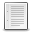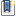File : Details

# varspace()

Memory allocated to specified variables, to containers components, or to results of expressions
Details
Version
1.0
Author
Samuel Gougeon
Maintainer
Samuel Gougeon
Categories
Supported Scilab Version
5.5
Creation Date
July 13, 2015Description
```            varspace() returns the number of bytes allocated to each variable specified by
its name or directly provided. When an heterogenous container like a list, a
cells array or a structure is provided, the vector or matrix of numbers of bytes
allocated to their components is returned.

varspace() will be useful to optimize algorithms using a lot of intermediate
memory, or simply to get aware about memory consumption w.r.t. to data types and
sizes.

Help page, examples and results follow.

--> varspace

Memory space taken by specified variables, litterals, or containers components

CALLING SEQUENCES
-----------------
varspace              // displays this help
varspace var1 var2 ...
vm = varspace("var1", "var2", ...)
Vm = varspace(VarNames)
Vm = varspace(obj#1)
VM = varspace(obj#1, obj#2, ...)

PARAMETERS
----------
var1, var2,.. :
Words = names of variables that's memory space must be
returned or displayed.
VarNames :
Vector or matrix of non-empty words = variables names
vm :   Row of decimal numbers = memory space allocated to associated
variables, in bytes.

Vm :   Vector or matrix of decimal numbers, having the size of
VarNames or obj#1.
Vm(i)   = memory allocated to the variable named VarNames(i)
or to the component obj#1(i).
Vm(i,j) = memory allocated to the variable named VarNames(i,j)
or to the component obj#1(i,j).

obj#1, obj#2,.. :
Literal objects, or variables of simple data type, or
heterogeneous containers (Lists, Cells arrays, Structures
arrays). If a component C is itself a container, varspace()
is not recursively applied to its components: Only its whole
varspace is returned at the C position.

VM :   List of vectors or matrices of decimal numbers.
VM(i) has the size of obj#i. It provides the vector or matrix
of numbers of bytes allocated to the components of obj#i:
- literal objects or expressions: the object or the result
of the expression is first assigned to a temporary variable.
The memory space taken by this one is returned.
- words or texts (scalar, vector or matrix): are considered
as objects in their own, not as names of variables to be
byte-sized.

DESCRIPTION
-----------
For a series of scilab variables, varspace() returns the respective
amounts of allocated memory, in bytes.
If a queried variable or a component of a list or stucture does
not exist, a memory amount == -1 is returned.
NOTE: Only local variables are seen. To make a global variable locally
visible, please apply global() to it before calling varspace().
NOTE: If two variables with the same name are defined in the local
and the global spaces and are queried, only the local one is
considered.

EXAMPLES
--------
clear d
a = 1, b = [1 2], c = [1 2 3], e = [], es = '', s1 = 'a', s2 = 'ab'
ec = cell(); ec22 = cell(2,2); ec44 = cell(4,4); ec88 = cell(8,8);

// Specifying names of variables
// -----------------------------
varspace ec ec22 ec44 ec88 // inline syntax (then without coma)
varspace('a,b ,c, d, e,es, s1,s2, ec22 ec88, a')
varspace(['a' 'b' 'c' ; 'd' 'ec' 'a']) // matrix of variables names

// Specifying objects
// ------------------
//  Matrix of texts considered as an object (because '' can't be the name
//   of a variable) :
varspace(['a' 'b' 'c' ; 'd' 'e' ''])

// Containers like a cells array: the memory allocated to each array's
//  component is returned:
c = makecell([2,3], %t,%pi,%z, list('hello',rand(3,5)),'',rand(1,3)<0.5)

// The memory allocated to the cell is greater than the sum of
//  memory allocated to its components:
varspace(c)
sum(varspace(c)), varspace("c")

// This is also the case for lists, that can have undefined components:
m = rand(100,100);
L = list(m, m+%i*m, , m<0.5, int8(m), int16(m), int32(m));
varspace('m', L)  // Since L is an object, 'm' is considered
//  as a simple string (another object),
//  instead of a variable's name
// To get the varspace of a litteral string, you may embed it in a list:
varspace(list("a,b ,c, d, e,es, s1,s2, ec22 ec88, a"))
// .. or priorly assign it to a variable:
s = "a,b ,c, d, e,es, s1,s2, ec22 ec88, a";
varspace s

* who : http://help.scilab.org/docs/current/en_US/who.html
* http://wiki.scilab.org/Memory%20representation%20of%20variables

RESULTS OF EXAMPLES
===================
--> clear d    // d does no longer exist
--> a = 1; b = [1 2]; c = [1 2 3]; e = []; es = ''; s1 = 'a'; s2 = 'ab';
--> ec = cell(); ec22 = cell(2,2); ec44 = cell(4,4); ec88 = cell(8,8);
-->
--> // Specifying names of variables
--> // -----------------------------
--> varspace ec ec22 ec44 ec88
ans  =
160.    240.    480.    1440.

--> varspace('a,b ,c, d, e,es, s1,s2, ec22 ec88, a')
ans  =
16.    32.    40.  - 1.    16.    32.    32.    32.    240.    1440.    16.

--> varspace(['a' 'b' 'c' ; 'd' 'ec' 'a'])
ans  =
16.    32.     40.
- 1.     160.    16.

-->// Specifying objects
-->// ------------------

--> varspace(['a' 'b' 'c' ; 'd' 'e' ''])
ans  =
64.

--> c=makecell([2,3],%t,%pi,%z,list('hello',rand(3,5)),'',rand(1,3)<0.5)
c  =
!%t        3.1415927  z           !
!                                 !
!{2 list}  ""         [%f,%t,%f]  !

--> varspace(c)
ans  =
24.     24.    56.
208.    32.    24.

--> sum(varspace(c)), varspace("c")
ans  =
368.
ans  =
552.

--> m = rand(100,100);
--> L = list(m, m+%i*m, , m<0.5, int8(m), int16(m), int32(m));
--> varspace('m', L)
ans  =
ans(1)
32.
ans(2)
80016.    160016.  - 1.    40016.    10024.    20024.    40024.
```Files (1)Miscellaneous file
```File defining the function varspace()

* MAKE IT AVAILABLE in EVERY SESSION: Click there to see indications

* Get help: Enter "varspace" without parameters

```News (0)Comments (0)Leave a comment
Login withEmail notifications